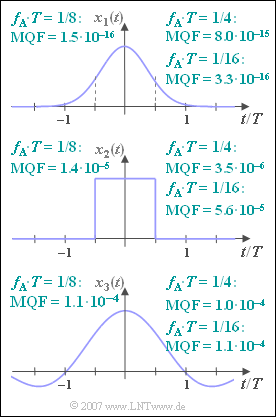# Exercise 5.3: Mean Square ErrorGaussian pulse, square pulse,
sinc pulse and some parameters

We consider three pulses, namely

• Gaussian pulse  with amplitude  $A$  and equivalent duration  $T$:
$$x_1(t) = A \cdot {\rm e}^{- \pi (t/T)^2} \hspace{0.05cm},$$
• rectangular pulse  $x_2(t)$  with amplitude  $A$  and (equivalent) duration  $T$:
$$x_2(t) = \left\{ \begin{array}{c} A \\ 0 \\ \end{array} \right.\quad \begin{array}{*{10}c} {\rm{f\ddot{u}r}} \\ {\rm{f\ddot{u}r}} \\ \end{array}\begin{array}{*{20}c} |t| < T/2 \hspace{0.05cm}, \\ |t| > T/2 \hspace{0.05cm}, \\ \end{array}$$
• a so called  "sinc pulse"  according to the following definition:
$$x_3(t) = A \cdot {\rm sinc}(t/ T) ,\hspace{0.15cm}{\rm sinc}(x) = \sin(\pi x)/(\pi x)\hspace{0.05cm}.$$

Let the signal parameters be  $A = 1\ {\rm V}$  and  $T = 1\ {\rm ms}$ in each case.

The conventional  Fourier transform  leads to the following spectral functions:

• $X_1(f)$  is also Gaussian,
• $X_2(f)$  runs according to the  $\rm sinc$ function,
• $X_3(f)$  is constant for  $|f| < 1/(2 T)$  and outside zero.

For all spectral functions,  $X(f = 0) = A \cdot T$.

If the discrete-frequency spectrum is determined by the  Discrete Fourier Transform  $\rm (DFT)$  with the DFT parameters

• $N = 512$   ⇒   number of samples considered in the time and frequency domain,
• $f_{\rm A}$   ⇒   interpolation distance in the frequency domain,

this will lead to distortions due to truncation and/or aliasing errors.

The other DFT parameters are clearly fixed withn  $N$  and  $f_{\rm A}$.  The following applies to these:

$$f_{\rm P} = N \cdot f_{\rm A},\hspace{0.3cm}T_{\rm P} = 1/f_{\rm A},\hspace{0.3cm}T_{\rm A} = T_{\rm P}/N \hspace{0.05cm}.$$

The accuracy of the respective DFT approximation is captured by the  "mean square error"  $\rm (MSE)$.
Here, we use the designation  $\rm MQF$   ⇒   (German:  "Mittlerer Quadratischer Fehler"):

$${\rm MQF} = \frac{1}{N}\cdot \sum_{\mu = 0 }^{N-1} \left|X(\mu \cdot f_{\rm A})-\frac{D(\mu)}{f_{\rm A}}\right|^2 \hspace{0.05cm}.$$

The resulting MQF values are given in the graph above, valid for  $N = 512$  as well as for

• $f_{\rm A} \cdot T = 1/4$,
• $f_{\rm A} \cdot T = 1/8$,
• $f_{\rm A} \cdot T = 1/16$.

Hints:

### Questions

1

Which range  $|f| \leq f_{\text{max}}$  is covered with  $N = 512$  and  $f_{\rm A} \cdot T = 1/8$ ?

 $f_{\text{max}} \cdot T\ = \$

2

At what time interval  $T_{\rm A}$  are the sampled values of  $x(t)$  available?

 $T_{\rm A}/T\ = \$

3

Due to which effect does the MQF value for the Gaussian pulse increase when using   $f_{\rm A} \cdot T = 1/4$  instead of  $f_{\rm A} \cdot T = 1/8$?

 The truncation error is significantly increased. The aliasing error is significantly increased.

4

Due to what effect does the MQF value for the Gaussian pulse increase when using  $f_{\rm A} \cdot T = 1/16$  instead of $f_{\rm A} \cdot T = 1/4$?

 The truncation error is significantly increased. The aliasing error is significantly increased.

5

Compare the  $\rm MQF$  values of the rectangular pulse  $x_2(t)$  with those of the Gaussian pulse  $x_1(t)$.  Which of the following statements are true?

 $\rm MQF$  becomes larger because the spectral function  $X_2(f)$  decays asymptotically slower than  $X_1(f)$. The aliasing error dominates. The truncation error dominates.

6

Compare the  $\rm MQF$  values of the "sinc pulse"  $x_3(t)$  with those of the Gaussian pulse  $x_1(t)$.  Which of the following statements are true?

 $\rm MQF$  becomes larger because the spectral function  $X_3(f)$  decays asymptotically slower than  $X_1(f)$. The aliasing error dominates. The truncation error dominates.

### Solution

#### Solution

(1)  With the DFT parameters  $N = 512$  and  $f_{\rm A} \cdot T = 1/8$  the following follows after multiplying the two quantities:

$$f_{\rm P} \cdot T = N \cdot (f_{\rm A} \cdot T) = 64.$$
• This covers the frequency range  $-f_{\rm P}/2 \leq f < +f_{\rm P}/2$:
$$f_{\rm max }\cdot T \hspace{0.15 cm}\underline{= 32}\hspace{0.05cm}.$$

(2)  The periodisation of the time function is based on the parameter  $T_{\rm P} = 1/f_{\rm A} = 8T$.

• The distance between two samples is therefore
$$T_{\rm A}/T = \frac{T_{\rm P}/T}{N} = \frac{8}{512}\hspace{0.15 cm}\underline{ = 0.015625}\hspace{0.05cm}.$$

(3)  Correct is the proposed solution 1   ⇒   increase of the truncation error:

• This measure simultaneously halves  $T_{\rm P}$  from  $8T$  to  $4T$ .
• Thus, only samples in the range  $–2T \leq t < 2T$ are taken into account, which increases the truncation error.
• The mean square error  $(\rm MQF)$  increases from  $0.15 \cdot 10^{-15}$  to  $8 \cdot 10^{-15}$ for the Gaussian pulse  $x_1(t)$,
• although the aliasing error actually decreases slightly by this measure.

(4)  Correct is the proposed solution 2   ⇒   increase of the aliasing error::

• By halving  $f_{\rm A}$  ⇒   $f_{\rm P}$  is also halved.
• As a result, the aliasing error becomes somewhat larger with a smaller truncation error at the same time.
• Overall, for the Gaussian pulse  $x_1(t)$, the mean square error  $(\rm MQF)$  increases from  $1.5 \cdot 10^{-16}$  to  $3.3 \cdot 10^{-16}$.

(5)  Proposed solutions 1 and 2 are correct:

• As can be seen from the graph, the last statement is not true in contrast to the first two.
• Due to the slow  ($\rm sinc$–shaped)  decay of the spectral function, the aliasing error dominates.
• The  $\rm MQF$ value at  $f_{\rm A} \cdot T = 1/8$  with  $1.4 \cdot 10^{-5}$  is therefore significantly larger than for the Gaussian pulse  $(1.5 \cdot 10^{-16})$.

(6)  Proposed solution 3 is correct:

• The spectral function  $X_3(f)$  here has a rectangular lead, so that the first two statements do not apply.
• On the other hand, a truncation error is unavoidable with this  $\rm sinc$–shaped time function.  This leads to the large  $\rm MQF$ values given.